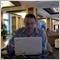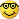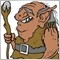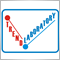# Moving Average - page 28Moderator
113445337

Available indicators shows the directions of a MA (up or down) ... in other words it just shows the slope as 1 or -1

however, it is useful to calculate the value of mathematical slope

or in mathematical language ... differentiation3582

These are not quite what I am looking for........... however, I did learn some things by reading of each one.......!500

???????

so what U all discuss is wants MA that colour such if heading down red up green?am I right?337

heartnet:
so what U all discuss is wants MA that colour such if heading down red up green?am I right?

No that is what I called as available indicators

Real mathematical slope is the degree between the MA and x axis

something like 'sin' and 'cos'3582

what I would like to have is something that says something like 'slope' or 'chop' depending on the degree of slope. appearing as a word on the chart... eg if the angle is less than 30. that would be chop and more than 30 would be trend, the number 30 is not necessarily the delimiter... just an idea.....5921

Blaiserboy:
what I would like to have is something that says something like 'slope' or 'chop' depending on the degree of slope. appearing as a word on the chart... eg if the angle is less than 30. that would be chop and more than 30 would be trend, the number 30 is not necessarily the delimiter... just an idea.....

Maybe this one: https://www.mql5.com/en/forum/1730566936

Hi,

I've finished new version of AllAverages_v2.1.

In this version I've used new MTF technique without calls of iCustom function.

So it's maybe interesting for other coders.

New list of MAs:

MA_Method= 0: SMA - Simple Moving Average

MA_Method= 1: EMA - Exponential Moving Average

MA_Method= 2: Wilder - Wilder Exponential Moving Average

MA_Method= 3: LWMA - Linear Weighted Moving Average

MA_Method= 4: SineWMA - Sine Weighted Moving Average

MA_Method= 5: TriMA - Triangular Moving Average

MA_Method= 6: LSMA - Least Square Moving Average (or EndPoint MA, Linear Regression Line, Modified MA)

MA_Method= 7: SMMA - Smoothed Moving Average

MA_Method= 8: HMA - Hull Moving Average by Alan Hull

MA_Method= 9: ZeroLagEMA - Zero-Lag Exponential Moving Average

MA_Method=10: DEMA - Double Exponential Moving Average by Patrick Mulloy

MA_Method=11: T3 - T3 by T.Tillson

MA_Method=12: ITrend - Instantaneous Trendline by J.Ehlers

MA_Method=13: Median - Median

MA_Method=14: GeoMean - Geometric Mean

MA_Method=15: REMA - Regularized EMA by Chris Satchwell

MA_Method=16: ILRS - Integral of Linear Regression Slope

MA_Method=17: IE/2 - Combination of LSMA and ILRS

As you can see I've excluded MMA from the list because it's similar to LSMA or Linear Regression Line.

Regards,

Igor

Files:301

Hi,

I've finished new version of AllAverages_v2.1.

In this version I've used new MTF technique without calls of iCustom function.

So it's maybe interesting for other coders.

New list of MAs:

MA_Method= 0: SMA - Simple Moving Average

MA_Method= 1: EMA - Exponential Moving Average

MA_Method= 2: Wilder - Wilder Exponential Moving Average

MA_Method= 3: LWMA - Linear Weighted Moving Average

MA_Method= 4: SineWMA - Sine Weighted Moving Average

MA_Method= 5: TriMA - Triangular Moving Average

MA_Method= 6: LSMA - Least Square Moving Average (or EndPoint MA, Linear Regression Line, Modified MA)

MA_Method= 7: SMMA - Smoothed Moving Average

MA_Method= 8: HMA - Hull Moving Average by Alan Hull

MA_Method= 9: ZeroLagEMA - Zero-Lag Exponential Moving Average

MA_Method=10: DEMA - Double Exponential Moving Average by Patrick Mulloy

MA_Method=11: T3 - T3 by T.Tillson

MA_Method=12: ITrend - Instantaneous Trendline by J.Ehlers

MA_Method=13: Median - Median

MA_Method=14: GeoMean - Geometric Mean

MA_Method=15: REMA - Regularized EMA by Chris Satchwell

MA_Method=16: ILRS - Integral of Linear Regression Slope

MA_Method=17: IE/2 - Combination of LSMA and ILRS

As you can see I've excluded MMA from the list because it's similar to LSMA or Linear Regression Line.

Regards,

Igor

Maybe next step you can put color change when the trend up and down.Like fxsniper ma.72

EMA Indicator

Is it possible to program an indicator that will display the current value of an MA on the chart in the form of a horizontal line rather than a squiggly line, It only needs to be at the cuurent price but move as the value changes. The object is simply to eliminate the squiggly line and replace it with a horizontal line at the current value of the MA.

With the MA period...Type MA and color adjustable.

Files:
ma_line.gif 22 kb